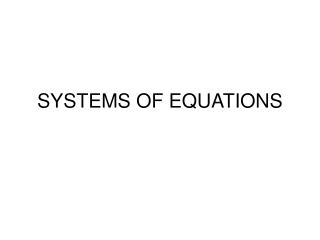DownloadDownload PresentationSYSTEMS OF EQUATIONS

# SYSTEMS OF EQUATIONS

Download Presentation## SYSTEMS OF EQUATIONS

- - - - - - - - - - - - - - - - - - - - - - - - - - - E N D - - - - - - - - - - - - - - - - - - - - - - - - - - -
##### Presentation Transcript

1. SYSTEMS OF EQUATIONS

2. Substitution Method Ex. 1: 2x + y = 15 y = 3x You now have to go back to one of your original equations where you will substitute the known value of x in order to determine the value of y We have been using the Substitution Property in our proofs. Now we are going to use substitution to solve systems of equations. 2x + 3x = 15 so 5x = 15 and x = 3 y = 3(3) = 9 Write your answer as an ordered pair (3, 9)

3. Ex. 2 3x – y = 13 y = x + 5 3x – ( x + 5 ) = 13 3x – x – 5 = 13 2x = 18 x = 9 Now substitute back into one of the original equations to find y. y = 9 + 5 = 14

4. You may have to rearrange one of the equations before you do the substitution. x + 4y = 20 2x + 3y = 10 Rearrange: x = 20 – 4y Now substitute and solve for y: 2 (20 – 4y)+3y=10 so 40 – 8y + 3y=10 -5y = -30 so y = 6 Find x: x + 4(6) = 20 x+ 24 = 20 x = -4

5. Solving systems of equations by adding or subtracting equations Example: x + 5y = -7 3x – 5y = 15 Now substitute into either equation to find y: 2 + 5y = -7 5y = -9 y = -9/5 If you add these two equations together, the y term drops out! 4x = 8 x = 2

6. Example: 4x – y = 16 3x – y = 11 x = 5 4(5) – y = 16 20 – y = 16 y = 4 (5, 4)

7. You may have to multiply one of the equations before you add or subtract: -3x + 2y = 10 2x – y = -2 Multiply this equation by 2 4x – 2y = -4 Now add the equations together x = 6 After substitution we find y = 14 (6,14)

8. You may have to multiply both of the equations by a number to get a variable to cancel out. 2x + 5y = 36 3x – 2y = -3 Multiply by 3 6x + 15y = 108 Multiply by 2 6x - 4y = -6 19y = 114 y= 6